Question

# The following sample observations were randomly selected: (Round the final answers to 4 decimal places.) X:...

The following sample observations were randomly selected: (Round the final answers to 4 decimal places.)

X: 16 14 9 17 12 11 20 18

Y: 14 21 1 13 11 16 14 15

a. Determine the 95% confidence interval for the mean predicted when X = 6.

b. Determine the 95% prediction interval for an individual predicted when X = 6.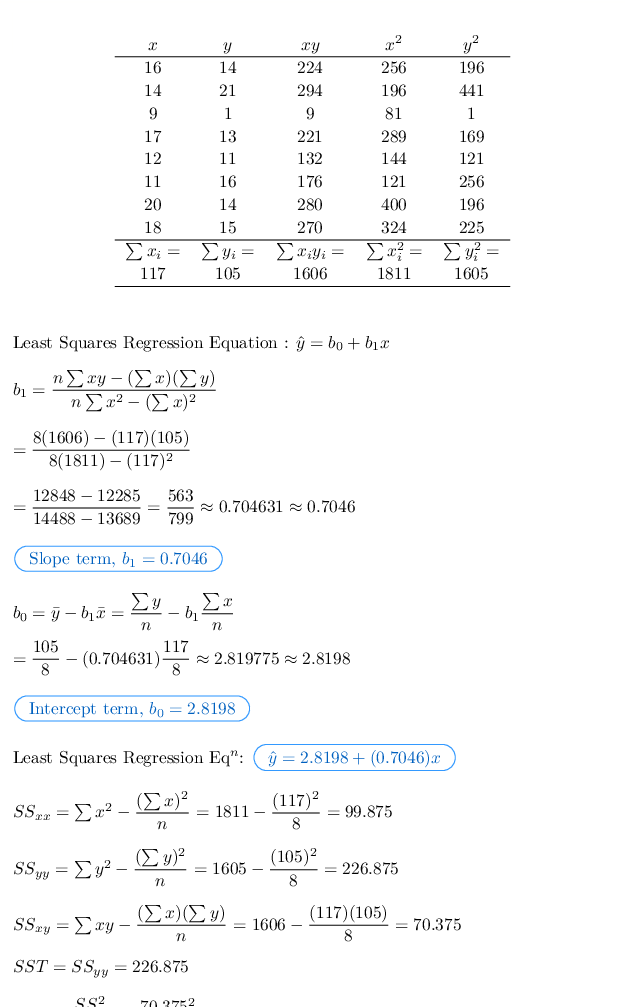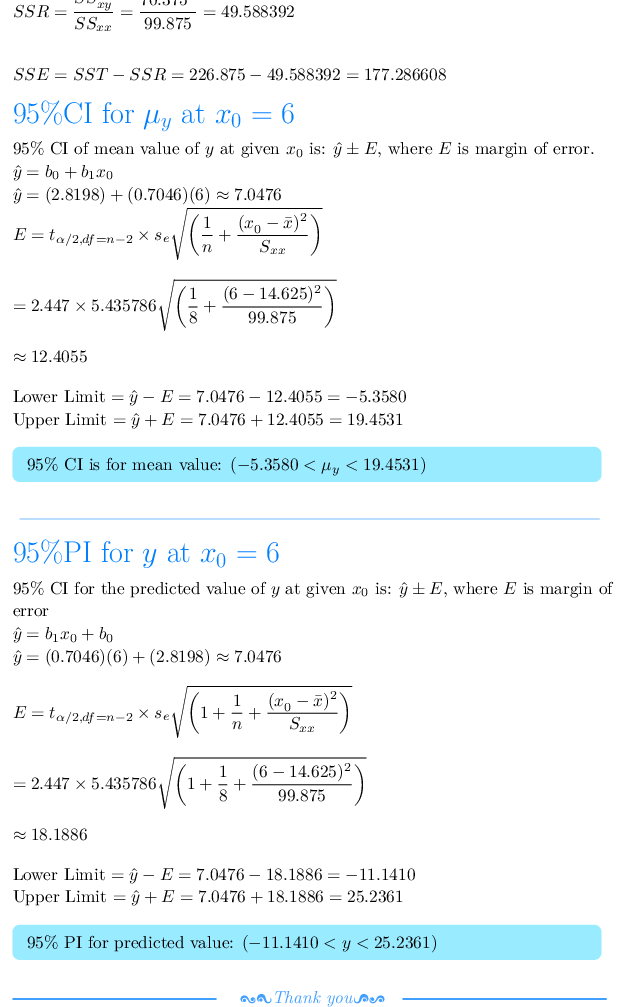#### Earn Coins

Coins can be redeemed for fabulous gifts.

Similar Homework Help Questions
• ### The following sample observations were randomly selected. (Do not round the intermediate values. Negative amount should...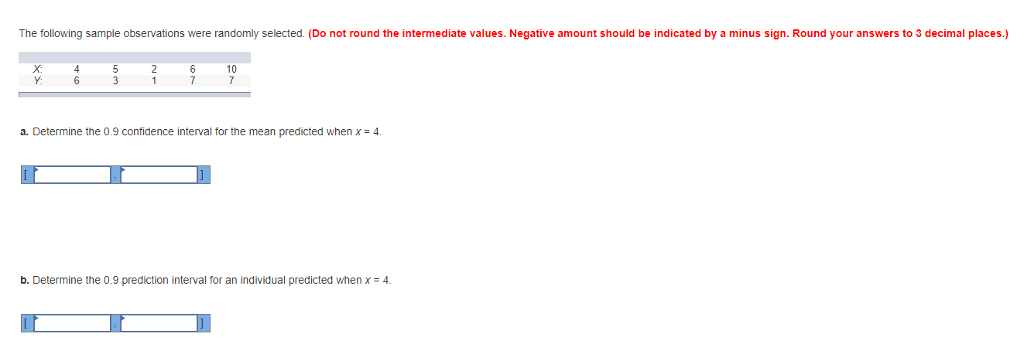The following sample observations were randomly selected. (Do not round the intermediate values. Negative amount should be indicated by a minus sign. Round your answers to 3 decimal places.) Y: a. Determine the 0.9 confidence interval for the mean predicted when x- 4 b. Determine the 0.9 prediction interval for an individual predicted whenx4

• ### The following sample observations were randomly selected. (Round intermediate calculations and final answers to 2 decimal...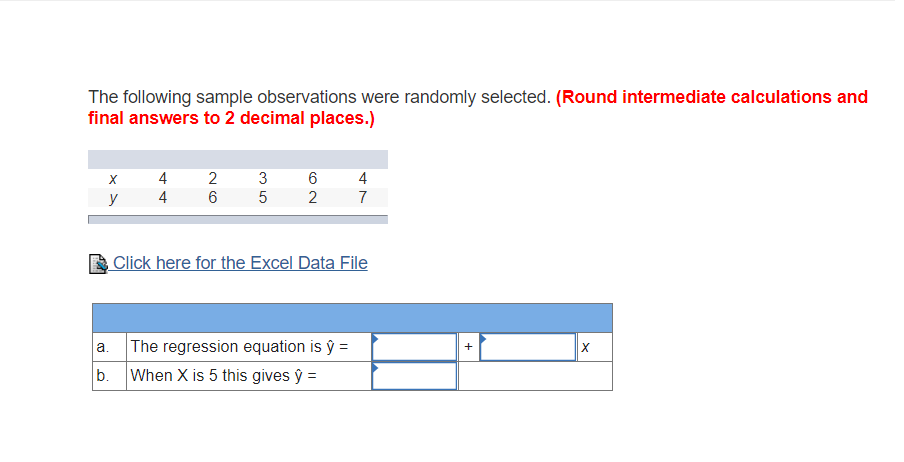The following sample observations were randomly selected. (Round intermediate calculations and final answers to 2 decimal places.) 4 6 4 &Click here for the Excel Data File a. The regression equation is y- When X is 5 this gives y =

• ### The following sample observations were randomly selected. (Round intermediate calculations and final answers to 2 decimal...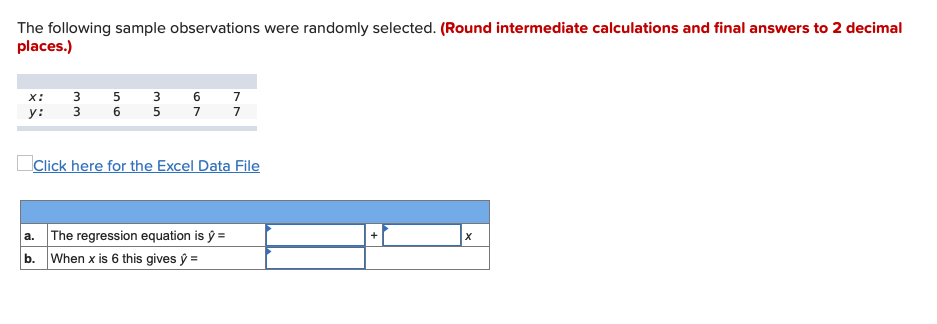The following sample observations were randomly selected. (Round intermediate calculations and final answers to 2 decimal places.) X: y : 3 3 5 6 3 5 6 7 7 7 Click here for the Excel Data File + a. The regression equation is ý = b. When x is 6 this gives y =

• ### The following sample observations were randomly selected. (Round your answers to 2 decimal places.) X: 4...

The following sample observations were randomly selected. (Round your answers to 2 decimal places.) X: 4 5 3 6 10 Y: 10.8 12.6 8 14.4 19.6 a. The regression equation is Yˆ Y⁢^⁢  = +  X b. When X is 7 this gives Yˆ Y⁢^⁢  =

• ### Given are five observations for two variables, x and y. (Round your answers to two decimal...

Given are five observations for two variables, x and y. (Round your answers to two decimal places.) xi 3 12 6 20 14 yi 55 40 55 10 15 (a) Estimate the standard deviation of ŷ* when x = 11. (b) Develop a 95% confidence interval for the expected value of y when x = 11. to (c) Estimate the standard deviation of an individual value of y when x = 11. (d) Develop a 95% prediction interval for y...

• ### A sample of 36 observations is selected from a normal population

1. A sample of 36 observations is selected from a normal population. The sample mean is 21 , and the population standards deviation is 5 . Conduct a test of hypothesis using the 0.05 significance level. Null hypothesis =20 . Alternate = ?2. A sample of 81 observations is taken from normal population with a standard deviation of 5 . The sample mean is 40 . Determine the 95 %confidence interval for the population.3. Given the following sample observations which...

• ### Exercise 2: The following sample observations were randomly selected. X Y 5 13 3 15 6...

Exercise 2: The following sample observations were randomly selected. X Y 5 13 3 15 6 7 3 12 4 13 4 11 6 9 8 5 a. Insert the trendline equation. b. Determine the coefficient of correlation and the coefficient of determination.

• ### Given are five observations for two variables, x and y. y3 7 6 11 14 Round...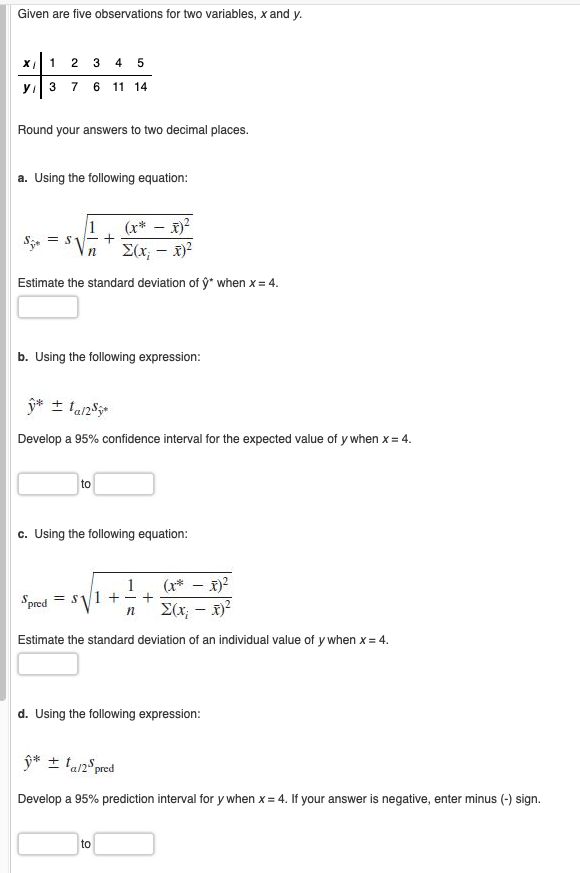Given are five observations for two variables, x and y. y3 7 6 11 14 Round your answers to two decimal places. a. Using the following equation: Estimate the standard deviation of ý" when x 4. b. Using the following expression: Develop a 95% confidence interval for the expected value of y when x-4. to c. Using the following equation: 1 nY Estimate the standard deviation of an individual value of y when x -4. d. Using the following expression...

• ### A sample of 23 observations is selected from a normal population where the population standard deviation...

A sample of 23 observations is selected from a normal population where the population standard deviation is 28. The sample mean is 71.   a. Determine the standard error of the mean. (Round the final answer to 3 decimal places.) The standard error of the mean is  . b. Determine the 95% confidence interval for the population mean. (Round the z-value to 2 decimal places. Round the final answers to 3 decimal places.) The 95% confidence interval for the population mean is...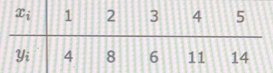Round your answers to two decimal places. a. Using the following equation:$$S_{\hat{y}},=s \sqrt{\frac{1}{n}+\frac{\left(x^{*}-\bar{x}\right)^{2}}{\sum\left(x_{i}-\bar{x}\right)^{2}}}$$ Estimate the standard deviation of $$\hat{y}^{*}$$ when $$x=3 .$$b. Using the following expression:$$\hat{y} * \pm t_{\alpha / 2} s_{\hat{y}}$$Develop a $$95 \%$$ confidence interval for the expected value of $$y$$ when $$x=3$$. toc. Using the following equation:$$s_{\text {pred }}=s \sqrt{1+\frac{1}{n}+\frac{\left(x^{*}-\bar{x}\right)^{2}}{\sum\left(x_{i}-\bar{x}\right)^{2}}}$$Estimate the standard deviation of an individual value of $$y$$ when $$x=3$$.d. Using the following expression:$$\hat{y}^{*} \pm t_{\alpha / 2} s_{\text {pred }}$$Develop a $$95 \%$$ prediction...